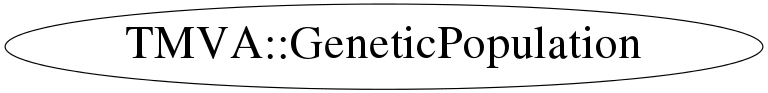# class TMVA::GeneticPopulation

```
Population definition for genetic algorithm

```

## Function Members (Methods)

public:
 virtual ~GeneticPopulation() void AddPopulation(TMVA::GeneticPopulation* strangers) void AddPopulation(TMVA::GeneticPopulation& strangers) static TClass* Class() TMVA::GeneticPopulation GeneticPopulation(const TMVA::GeneticPopulation&) TMVA::GeneticPopulation GeneticPopulation(const vector& ranges, Int_t size, UInt_t seed = 0) Double_t GetFitness() const const vector& GetGenePool() const vector& GetGenePool() TMVA::GeneticGenes* GetGenes(Int_t index) Int_t GetPopulationSize() const const vector& GetRanges() const vector& GetRanges() void GiveHint(vector& hint, Double_t fitness = 0) virtual TClass* IsA() const void MakeChildren() void MakeCopies(int number) void Mutate(Double_t probability = 20, Int_t startIndex = 0, Bool_t near = kFALSE, Double_t spread = 0.10000000000000001, Bool_t mirror = kFALSE) void NextGeneration() TMVA::GeneticPopulation& operator=(const TMVA::GeneticPopulation&) void Print(Int_t untilIndex = -1) void Print(ostream& out, Int_t utilIndex = -1) void SetRandomSeed(UInt_t seed = 0) virtual void ShowMembers(TMemberInspector& insp) const void Sort() virtual void Streamer(TBuffer&) void StreamerNVirtual(TBuffer& ClassDef_StreamerNVirtual_b) void TrimPopulation() vector VariableDistribution(Int_t varNumber) TH1F* VariableDistribution(Int_t varNumber, Int_t bins, Int_t min, Int_t max)
private:
 TMVA::MsgLogger& Log() const TMVA::GeneticGenes MakeSex(TMVA::GeneticGenes male, TMVA::GeneticGenes female)

## Data Members

private:
 vector fGenePool the "genePool" where the individuals of the current generation are stored TMVA::MsgLogger* fLogger message logger Int_t fPopulationSizeLimit TRandom3* fRandomGenerator random Generator for this population vector fRanges contains the ranges inbetween the values of the coefficients have to be

## Class Charts## Function documentation

GeneticPopulation(const vector<TMVA::Interval*>& ranges, Int_t size, UInt_t seed = 0)
``` Constructor
```

``` destructor
```
void SetRandomSeed(UInt_t seed = 0)
``` the random seed of the random generator
```
void MakeCopies(int number)
``` produces offspring which is are copies of their parents
Parameters:
int number : the number of the last individual to be copied

```
void MakeChildren()
``` does what the name says,... it creates children out of members of the
current generation
children have a combination of the coefficients of their parents

```
TMVA::GeneticGenes MakeSex(TMVA::GeneticGenes male, TMVA::GeneticGenes female)
``` this function takes two individuals and produces offspring by mixing (recombining) their
coefficients

```
void Mutate(Double_t probability = 20, Int_t startIndex = 0, Bool_t near = kFALSE, Double_t spread = 0.10000000000000001, Bool_t mirror = kFALSE)
``` mutates the individuals in the genePool
Parameters:
double probability : gives the probability (in percent) of a mutation of a coefficient
int startIndex : leaves unchanged (without mutation) the individuals which are better ranked
than indicated by "startIndex". This means: if "startIndex==3", the first (and best)
three individuals are not mutaded. This allows to preserve the best result of the
current Generation for the next generation.
Bool_t near : if true, the mutation will produce a new coefficient which is "near" the old one
(gaussian around the current value)
double spread : if near==true, spread gives the sigma of the gaussian
Bool_t mirror : if the new value obtained would be outside of the given constraints
the value is mapped between the constraints again. This can be done either
by a kind of periodic boundary conditions or mirrored at the boundary.
(mirror = true seems more "natural")

```
TMVA::GeneticGenes* GetGenes(Int_t index)
``` gives back the "Genes" of the population with the given index.

```
void Print(Int_t untilIndex = -1)
``` make a little printout of the individuals up to index "untilIndex"
this means, .. write out the best "untilIndex" individuals.

```
void Print(ostream& out, Int_t utilIndex = -1)
``` make a little printout to the stream "out" of the individuals up to index "untilIndex"
this means, .. write out the best "untilIndex" individuals.

```
TH1F* VariableDistribution(Int_t varNumber, Int_t bins, Int_t min, Int_t max)
``` give back a histogram with the distribution of the coefficients
parameters:
int bins : number of bins of the histogram
int min : histogram minimum
int max : maximum value of the histogram

```
vector<Double_t> VariableDistribution(Int_t varNumber)
``` gives back all the values of coefficient "varNumber" of the current generation

```
``` add another population (strangers) to the one of this GeneticPopulation
```
``` add another population (strangers) to the one of this GeneticPopulation
```
void TrimPopulation()
``` trim the population to the predefined size
```
void GiveHint(vector<Double_t>& hint, Double_t fitness = 0)
``` add an individual (a set of variables) to the population
if there is a set of variables which is known to perform good, they can be given as a hint to the population
```
void Sort()
``` sort the genepool according to the fitness of the individuals
```
GeneticPopulation(const vector<TMVA::Interval*>& ranges, Int_t size, UInt_t seed = 0)
Int_t GetPopulationSize() const
`{ return fGenePool.size(); }`
Double_t GetFitness() const
`{ return fGenePool.size()>0? fGenePool.GetFitness() : 0; }`
const std::vector<TMVA::GeneticGenes>& GetGenePool() const
`{ return fGenePool; }`
const std::vector<TMVA::GeneticRange*>& GetRanges() const
`{ return fRanges; }`
std::vector<TMVA::GeneticGenes>& GetGenePool()
`{ return fGenePool; }`
std::vector<TMVA::GeneticRange*>& GetRanges()
`{ return fRanges; }`
void NextGeneration()
`{}`